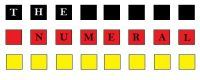# Repetition numeral | Multiplicative numeral | Fractional numeral in GermanThe repetition numeral.

Wiederholungszahlwörter.

There are two categorie of repetition numerals in german language:

- definite repetition numerals (bestimmter Wiederholungszahlwörter)

- indefinite repetition numerals (unbestimmter Wiederholungszahlwörter)

Translation of definite repetition numerals: once, twice, thrice... etc.

Indefinite repetition numerals are translated like this: sometimes, many times, more times etc.

The repetition numeral is formed with the help of the ending "-mal"

Examples:

1.   einmal (once)

2.   zweimal (twice)

3.   dreimal (thrice)

...

12. zwölfmal (twelve times)

etc.

If we add to the repetition numeral the ending "-ig" we will get an adjective:

1.   einmalig (one of a kind)

2.   zweimalig (two of a kind)

The multiplicative numeral

Vervielfältigungszahlwörter (Multiplikativa)

The multiplicative numeral expresses the proportional growth of an object or action.

Examples: twofold, threefold, tenfold etc.

The forming of the multiplicative numeral is realised with the help of the the ending "-fach".

Examples:

2. zweifach (twofold)

3. dreifach (threefold)

10. zehnfach (tenfold); 100. hundertfach (hundredfold)

etc.

Remember: the multiplicative numerals answer the question: wievielfach?

The fractional numeral

Der Bruckzahler

The fractional numeral shows one or more parts of the whole.

They are formed by adding the ending "-el" to the distributive numeral

Examples:

- halb = half
- drittel = one-third
- viertel = one-fourth
- dreivirtel = three-fourths

Other Examples:

- 1 1/2 = ein einhalb (one half)

- 2 1/5 = zwei einhalb (two one-fifth)

- 3 1/3 = drei eindrittel (three one-third)

- 1 5/6 = eins fünfsechstel (one five-sixth)

etc.

The distributive numeral

Die Verteilungszahlen

The distributive numeral defines a group by numbering.

It is formed with the help of two words: "zu" + the cardinal numeral with the ending "-t"

Examples:

- zu zweit (by two)

- zu dritt (by three)

- zu viert (by four)

etc.